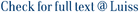The Lempel-Ziv factorization (LZ77) and the Run-Length encoded Burrows-Wheeler Transform (RLBWT) are two important tools in text compression and indexing, being their sizes z and r closely related to the amount of text self-repetitiveness. In this paper we consider the problem of converting the two representations into each other within a working space proportional to the input and the output. Let n be the text length. We show that RLBW T can be converted to LZ77 in O(n log r) time and O(r) words of working space. Conversely, we provide an algorithm to convert LZ77 to RLBW T in O n(log r + log z) time and O(r + z) words of working space. Note that r and z can be constant if the text is highly repetitive, and our algorithms can operate with (up to) exponentially less space than naive solutions based on full decompression.

From LZ77 to the run-length encoded burrows-wheeler transform, and back / Policriti, Alberto; Prezza, Nicola. - 28th Annual Symposium on Combinatorial Pattern Matching, CPM 2017, Leibniz International Proceedings in Informatics, LIPIcs, (2017), pp. 1-10. (28th Annual Symposium on Combinatorial Pattern Matching, CPM 2017, Polonia, July 4-6, 2017). [10.4230/LIPIcs.CPM.2017.17].

### From LZ77 to the run-length encoded burrows-wheeler transform, and back

#### Abstract

The Lempel-Ziv factorization (LZ77) and the Run-Length encoded Burrows-Wheeler Transform (RLBWT) are two important tools in text compression and indexing, being their sizes z and r closely related to the amount of text self-repetitiveness. In this paper we consider the problem of converting the two representations into each other within a working space proportional to the input and the output. Let n be the text length. We show that RLBW T can be converted to LZ77 in O(n log r) time and O(r) words of working space. Conversely, we provide an algorithm to convert LZ77 to RLBW T in O n(log r + log z) time and O(r + z) words of working space. Note that r and z can be constant if the text is highly repetitive, and our algorithms can operate with (up to) exponentially less space than naive solutions based on full decompression.
##### Scheda breve Scheda completa Scheda completa (DC)2017
9783959770392
Burrows-Wheeler transform; Compressed computation; Lempel-Ziv; Repetitive text collections; Software
File in questo prodotto:
File
p19-Policriti.pdf

Open Access

Tipologia: Versione dell'editore
Licenza: Creative commons
Dimensione 460.35 kB
Utilizza questo identificativo per citare o creare un link a questo documento: `https://hdl.handle.net/11385/194109`
•8
•ND